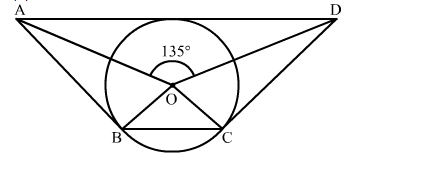# In the given figure, ∠AOD = 135∘ then ∠BOC is equal to`
Question:

In the given figure, ∠AOD = 135 then ∠BOC is equal to
(a) 25
(b) 45
(c) 52.5
(d) 62.5Solution:

We know that the sum of angles subtended by opposite sides of a quadrilateral having a circumscribed circle is 180 degrees.
∴∠AOD + ∠BOC =  180
⇒∠BOC =  180 − 135 = 45
Hence, the correct answer is option (b).